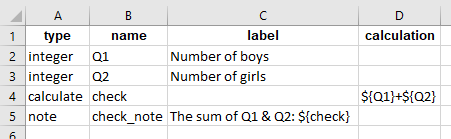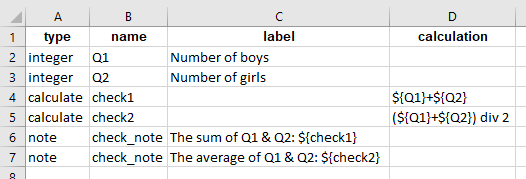# How do we use the calculate function in KoBoToolbox to add 2 variables?

How can I calculate the total number of the students (boy+girl) automatically? That means when I input boy and girl numbers, then automatically calculate the total students on the “total” field. Please see the screenshot:

2 Likes

I have seen it before, But not understand anything. It’s very advanced and calculated on excel sheet. But I only want that simple calculation on kobotoolbox form. Would you help me?

1 Like

Thanks a lot. It’s work perfectly. But I need to show the result on the input value, not the title. Is it possible? Just like this:

Hi @tanzilhuda,

Welcome to the community! Backing up with what @azadkhan has advised, you could also do the same as shown in the image below:

In the survey sheet of your xlsform:Reference xlsform:

GOOD DAY!

Thanks a lot. If I want to average of the summation? Then what code should I write?

Hi @tanzilhuda,

You could calculate the average as shown in the image below:

In the survey sheet of your xlsform:Reference xlsform: